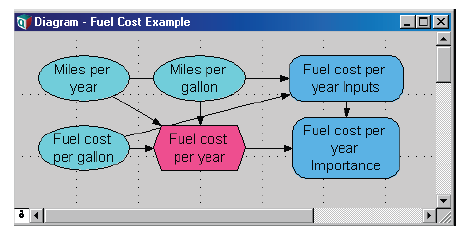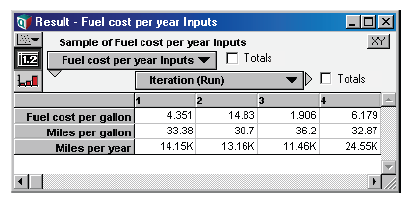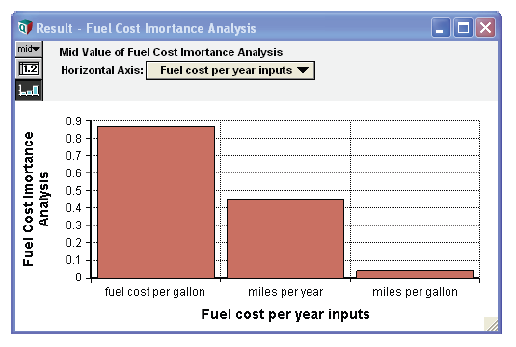# Importance analysis

Release: 4.6  •  5.0  •  5.1  •  5.2  •  5.3  •  5.4  •  6.0  •  6.1  •  6.2  •  6.3  •  6.4

In a model with uncertain variables, you might want to know how much each uncertain input contributes to the uncertainty in the output. Typically, a few uncertain inputs are responsible for the lion’s share of the uncertainty in the output, while the rest have little impact. You can then concentrate on getting better estimates or building a more detailed model for the one or two most important inputs without spending considerable time investigating issues that turn out not to matter very much.

The importance analysis features in Analytica can help you quickly learn which inputs contribute the most uncertainty to the output.

What is importance? This analysis uses as a metric of the “importance” of each uncertain input to a selected output, the absolute rank-order correlation between each input sample and the output sample. It is a robust measure of the uncertain contribution because it is insensitive to extreme values and skewed distributions. Unlike commonly used deterministic measures of sensitivity, such as used in the Tornado analysis, it averages over the entire joint probability distribution. Therefore, it works well even for models where there are strong interactions, where the sensitivity to one input depends on the value of another.

Create an importance variable:

1. Be sure you are in edit mode, viewing a Diagram window. Select an output variable, U, that depends on two or more uncertain inputs — possibly, an objective.
2. Select Make Importance from the Object menu.

If the selected output is U, it creates an index U_Inputs, a list of the uncertain inputs, and a general variable, U Importance, containing the importance of those inputs to the output.

Example:

Variable Miles_per_year := Triangular(1, 12K, 30K)
Variable Fuelcost_per_gallon := Lognormal(3)
Variable Miles_per_gallon := Normal(33, 2)
Variable Fuel_cost_per_year := (Fuel_cost_per_gallon*Miles_per_year)/ Miles_per_gallon

After you select Fuel_cost_per_year and then Make Importance from the Object menu, the diagram contains two new variables.Fuel cost per year Inputs is defined as a list identifiers, containing all the chance variable ancestors of the output node. It evaluates to an array of probability distributions, one for each chance variable. This array is self-indexed, with the index values consisting of handles to each input variable.Fuel cost per year Importance is defined as:

Abs(Rankcorrel(Fuel_cost_per_year_inputs, Fuel_cost_per_year, resultIfNoVariation:0))

The Rankcorrel function computes the rank-order correlation of each input to the output, and then the Abs function computes the absolute value, yielding a positive relative importance.As expected, Fuelcost_per_gallon contributes considerably more uncertainty to Fuel_cost_per_year than Miles_per_gallon.

Tip

Importance, like every other statistical measure, is estimated from the random sample. The estimates can vary slightly from one computation to another due to random noise. For a sample size of 100, an importance of 0.1 might not be significantly different from zero. But an importance of 0.5 is significantly different from zero. The main goal is to identify two or three that are the primary contributors to the uncertainty in the output. For greater precision, use a larger sample size

Updating inputs to importance analysis: If you create an importance analysis variable for U, and later add or remove uncertain variables that affect U, the uncertainty analysis is not automatically updated to reflect those changes. You can update the analysis either by:

• Select U and then select Make Importance from the Object menu. It automatically updates the importance analysis to reflect any new or removed uncertain inputs.
• Draw an arrow from any new uncertain input into index U inputs. It adds the new variable as an uncertain input. Similarly, you can remove a variable from U inputs by redrawing an arrow from that variable into U inputs.